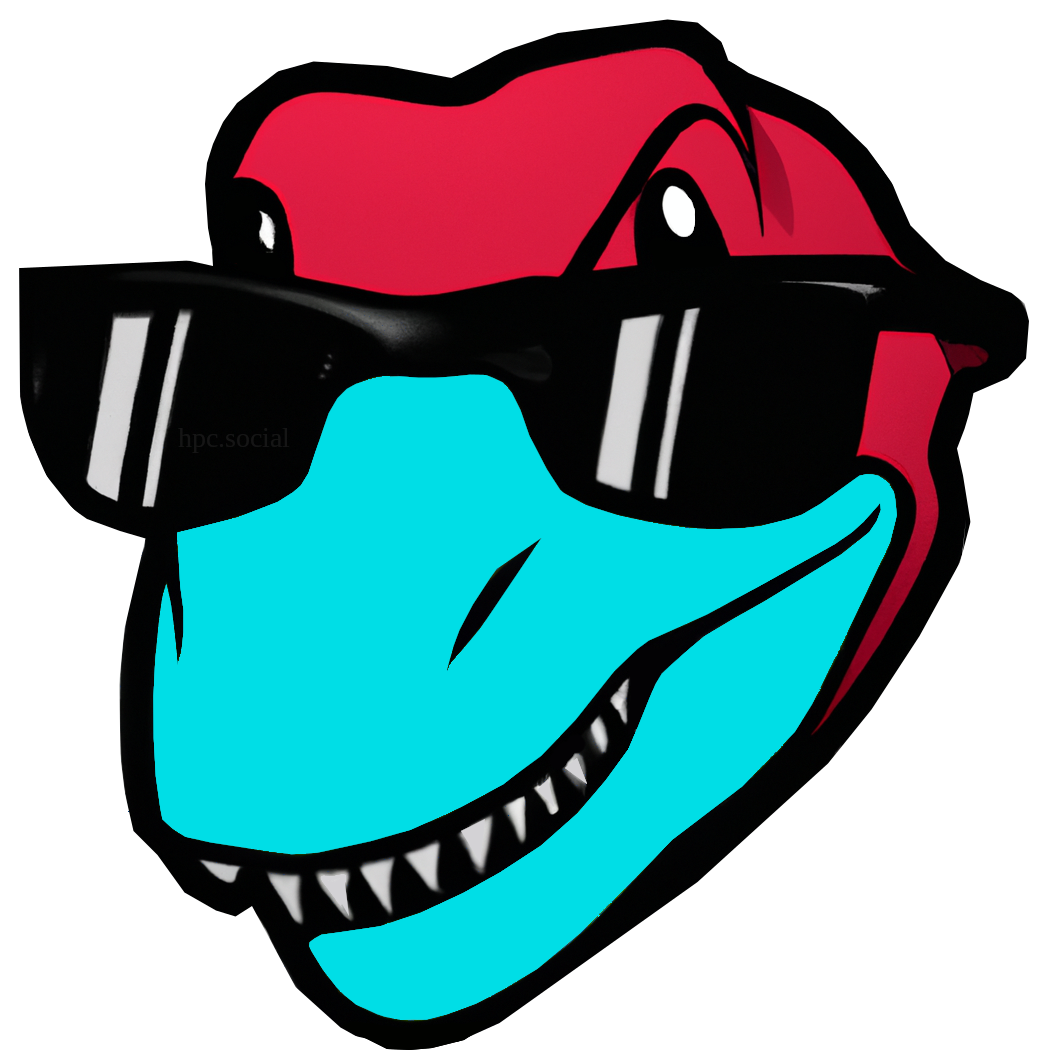This is a crosspost from   Cleve’s Corner: Cleve Moler on Mathematics and Computing Cleve Moler is the author of the first MATLAB, one of the founders of MathWorks, and is currently Chief Mathematician at the company. He writes here about MATLAB, scientific computing and interesting mathematics.. See the original post here.

# R2-D2, Rotations and Dilations in Two Dimensions

R2_D2 is is the name I've given a new MATLAB program that provides animations of 2-by-2 rotation and dilation matrices. I admit I chose "dilations" so the acronym would be memorable, but otherwise the code has little to do with the famous Star Wars droid.

### Contents

#### House

This outline of a house is featured in Experiments with MATLAB. The data are the 11 blue dots. The coordinates of each dot form a 2-by-1 vector; the 2-by-2 rotation and dilation matrices multiply each of these vectors separately. (The lines between the dots complete the picture and are not involved in any computation.)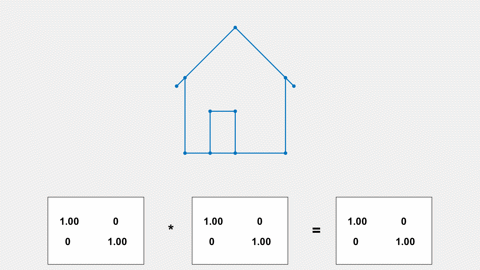The house also appears in several editions of Gil Strang's textbooks. The cover of the third edition of Strang's Introduction to Linear Algebra features nine houses on a quilt made by Gil's friend Chris Curtis.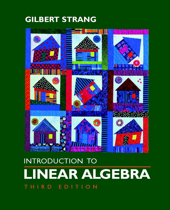#### Hand

This outline of a hand, which I made from measurements of my own hand, is also used in Experiments with MATLAB. There are 37 points, so Hand is a 2-by-37 matrix.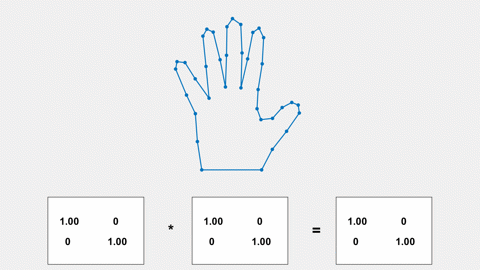#### Rotation

Multiplication by this matrix produces a two-dimensional rotation by an angle theta.

$$R = \left( \begin{array}{rr} \cos{\theta} & \sin{\theta} \\ - \sin{\theta} & \cos{\theta} \end{array} \right)$$

R is displayed in the first panel. If the house is not rotating in your browser, try this link: https://blogs.mathworks.com/cleve/files/house_rotate.gif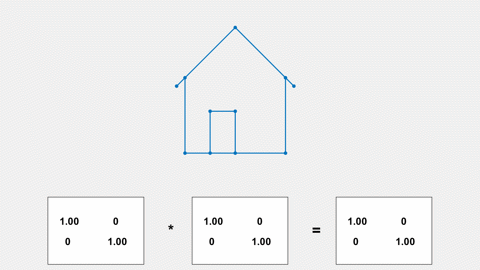#### Dilation

Dilation is the process of making objects larger or smaller. Multiplication by this diagonal matrix produces a dilation by a factor sigma.

$$S = \left( \begin{array}{rr} \sigma & 0 \\ 0 & \sigma \end{array} \right)$$

S is displayed in the second panel. The animation is also available at: https://blogs.mathworks.com/cleve/files/hand_dilate.gif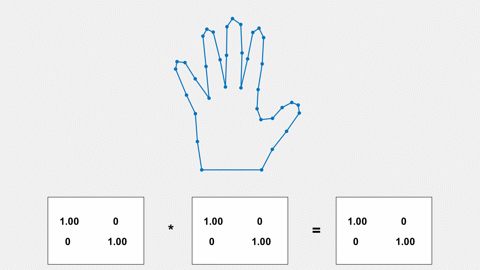#### Both

Here rotation and dilation are combined. The product of the rotation and dilation matrices drives the action. If you are missing all the action, try: https://blogs.mathworks.com/cleve/files/hand_both.gif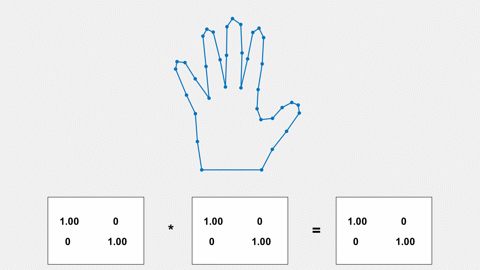#### R2_D2

When you run R2_D2 on your own computer, you can drive the rotations and dilations yourself. Mousing outside of the object creates rotation and mousing inside produces dilation.

The R2_D2 program is available from https://blogs.mathworks.com/cleve/files/R2_D2_4.m.